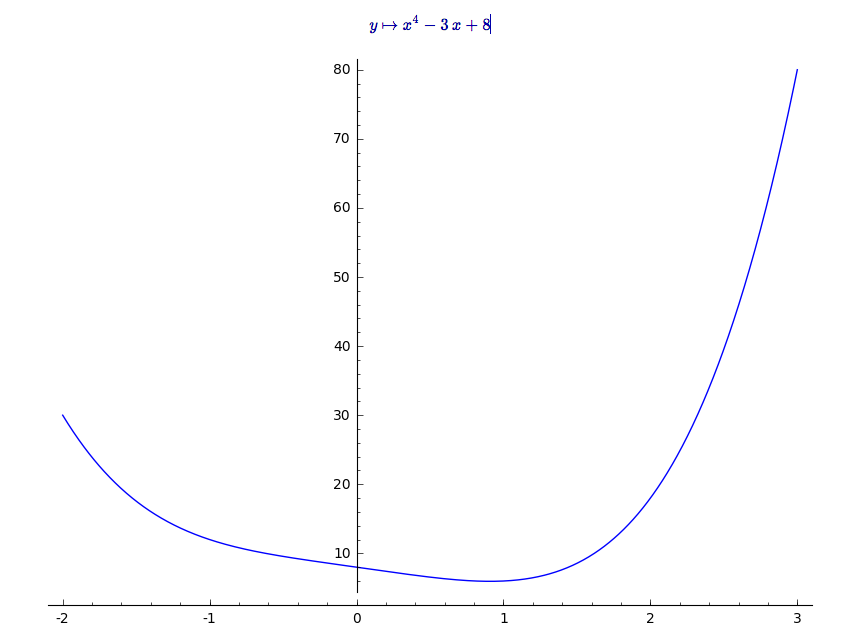# Numerical real solution of derivative

I would like to know where a function attains its maximum, so I'm trying to solve some

diff(y,x),x

where y depends on y. I have difficulties with Sage returning equations, complex roots, converting equations, find_maximum_on_interval command etc, and instead of spending another hour trying to figure it out myself, I thought I would try asking here...

Here is a more concrete example:

f = log(2.02 * x + 1) / 2 + log( -2 * x + 1) / 2

find_local_maximum(diff(f,x), 0, 1)

What is mysterious for me, is that the above works if instead I have

f = log(1.01 * x + 1) / 2 + log( -x + 1) / 2

edit retag close merge delete

It would make it easier to understand your problem, and to provide an answer, if you could provide a reproducible example, i.e. enough code to reproduce the equations, complex roots, and converting equations you mention. This would also increase the value of both question and answer for others who might face similar a challenge in the future.

Check this. It seems to work fine. If you need precision it is possible you need to use octave or matlab instead of sage.

The same issue persists - changing the variable x to 2x shouldn't make a difference, but it does. This might not even be an issue with precision, very weird.

Sort by » oldest newest most voted

Ok, I see what is happening. The derivative of your function (the example that you put) is not bounded on the interval $(0,1)$, i.e. it is not defined in the points $-1/2$ and $1/2$ where it is going to infinity.

But sage works fine, it said that the maximum is in $1/2$ because it is an asymptote. In other words

$$\lim_{x\to\frac12^-}\frac{\mathrm d}{\mathrm dx}\left(\frac12(\log(2.02x + 1) + \log( -2x + 1) )\right)=+\infty$$

and

$$\lim_{x\to\frac12^+}\frac{\mathrm d}{\mathrm dx}\left(\frac12(\log(2.02x + 1) + \log( -2x + 1) )\right)=-\infty$$

Check this:

http://www.wolframalpha.com/input/?i=limit+diff(log(2.02+*+x+%2B+1)+%2F+2+%2B+log(+-2+*+x+%2B+1)+%2F+2,x)+when+x-%3E1%2F2


So, Sage is not doing wrong... it is doing the "correct" answer. Well, the correct answer will be to say that there is no maximum in the interval, because the function is not bounded.

But if you change your interval by $(0,1/2)$ then you get the answer of $\approx 0.01$, check this. I tested in all the other major CAS with the same both results ;)

P.S.: the maximum in the interval $(0,1/2)$ is exactly $0.01$. It would be interesting to see how to get this answer directly. From mathematica I get something similar to sage, but maple and wolframalpha let me exactly the correct value. I will test geogebra now.

P.S2: geogebra found the exact value too.

more

1

Note that find_local_maximum is explicitly a numerical computation, not an exact solution.

@kcrisman, yes, I noticed. What is fun is that mathematica do the same thing: a numerical computation. But a lazy search on wolframalpha does the exact solution, i.e. a symbolic solution... LOL.

@Masacroso in this regard WolframAlpha might prove to be a bit like MS Word. It's easy at first, but down the road, what at first seemed to be a convenience is now a bug.

Would this give you what you are looking for? (assuming your interval is [-1;2]

f(y) = x^4-3*x+8
f.show()
find_local_maximum(f, -1, 2)


I am generally big on plotting unction like this, you can try to get a feel of your function

plot(f(y), -2,3)I highly recommend the SageMath Calculus Tutorial if you at all have the time.

### Update,

If I run your updated model with your coefficients, e.i.,

f = (1/4)*log(2.02000000000000*x + 1) + (1/2)*log(0.00999999999999979*x + 1) + (1/4)*log(-2*x + 1)
find_local_maximum(diff(f,x), 0, 1)


i get,

(95160969.23167175, 0.50000000262712752)


Could you explain what you expect and why? Did i possibly misinterpret your code? (I did added some parenthesis and some multiplication symbols).

more

Thanks for your help again, I've again updated my answer. It seems that 2*x and x makes all the difference for Sage. The solution should be some x around 0.01.